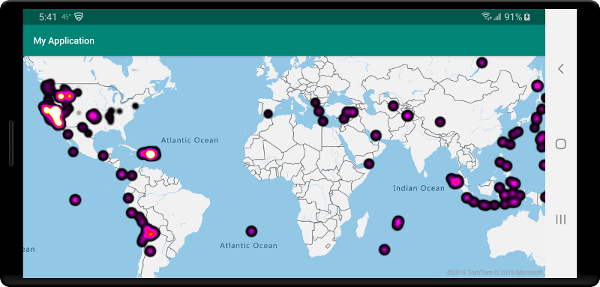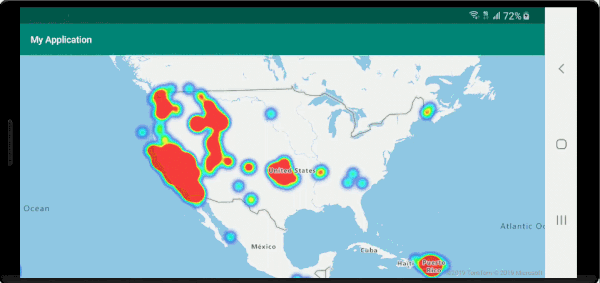# 添加热度地图层 (Android SDK)

• 温度数据：提供两个数据点之间的温度近似值。
• 噪声传感器数据：不仅显示传感器所在位置的噪声强度，而且还可提供一段距离内的消散情况见解。 任何一个场地的噪声级别可能不高。 如果多个传感器的噪声覆盖区域重叠，则此重叠区域可能会出现更高的噪声级别。 因此，重叠区域将在热度地图中可见。
• GPS 轨迹：将速度包含为加权高度地图，其中每个数据点的强度基于速度。 例如，使用此功能可以查看车辆的加速位置。

## 添加热度地图层

``````//Create a data source and add it to the map.
DataSource source = new DataSource();

//Import the geojson data and add it to the data source.
source.importDataFromUrl("https://earthquake.usgs.gov/earthquakes/feed/v1.0/summary/all_week.geojson");

//Add data source to the map.

//Create a heat map layer.
HeatMapLayer layer = new HeatMapLayer(source,
heatmapOpacity(0.8f)
);

//Add the layer to the map, below the labels.
``````
``````//Create a data source and add it to the map.
val source = DataSource()

//Import the geojson data and add it to the data source.
source.importDataFromUrl("https://earthquake.usgs.gov/earthquakes/feed/v1.0/summary/all_week.geojson")

//Add data source to the map.

//Create a heat map layer.
val layer = HeatMapLayer(
source,
heatmapOpacity(0.8f)
)

//Add the layer to the map, below the labels.
``````## 自定义热度地图层

• `heatmapRadius`：定义要呈现每个数据点的像素半径。 可将半径设置为固定数字或表达式。 使用表达式可以根据缩放级别来缩放半径，并在地图上表示一个一致的空间区域（例如，5 英里半径）。

• `heatmapColor`：指定如何为热度地图赋色。 颜色渐变是热度地图的常用功能。 可以使用 `interpolate` 表达式来实现该效果。 还可以使用 `step` 表达式为热度地图赋色，直观地将密度分解到类似于等高线或雷达式地图的范围中。 这些调色板定义了从最小到最大密度值的颜色。

将热度地图的颜色值指定为基于 `heatmapDensity` 值的表达式。 “内插”表达式的索引 0 处定义了没有数据的颜色区域，或“递阶”表达式的默认颜色。 可以使用此值来定义背景色。 通常此值设置为“透明”，或“半透明的黑色”。

下面是颜色表达式示例：

内插颜色表达式 递阶色表达式
interpolate(
linear(),
heatmapDensity(),
stop(0, color(Color.TRANSPARENT)),
stop(0.01, color(Color.MAGENTA)),
stop(0.5, color(parseColor("#fb00fb"))),
stop(1, color(parseColor("#00c3ff")))
)`
step(
heatmapDensity(),
color(Color.TRANSPARENT),
stop(0.01, color(parseColor("#000080"))),
stop(0.25, color(parseColor("#000080"))),
stop(0.5, color(Color.GREEN)),
stop(0.5, color(Color.YELLOW)),
stop(1, color(Color.RED))
)
• `heatmapOpacity`：指定热度图层的不透明或透明程度。

• `heatmapIntensity`：对每个数据点的权重应用乘数，以增加热度地图的整体强度。 这会导致数据点的权重出现差异，使其更易于可视化。

• `heatmapWeight`：默认情况下，所有数据点的权重均为 1，并且是平均加权的。 权重选项充当乘数，可以设置为数字或表达式。 如果将某个数字设置为权重，则这相当于将每个数据点置于地图上两次。 例如，如果权重为 `2`，则密度会加倍。 将权重选项设置为一个数字，以类似于使用强度选项的方式来呈现热度地图。

但是，如果使用表达式，则每个数据点的权重可以基于每个数据点的属性。 例如，假设每个数据点表示地震。 震级值是每个地震数据点的重要指标。 地震时时刻刻都在发生，但大多数震级都很低，以致于感觉不到。 在表达式中使用震级值可将权重分配到每个数据点。 使用震级值分配权重可以更好地在热度地图中表示地震的严重程度。

• `minZoom``maxZoom`：应显示层的缩放级别范围。

• `filter`：用于限制从源检索并在层中呈现的筛选器表达式。

• `sourceLayer`如果连接到层的数据源是矢量图块源，那么必须指定矢量图块中的源层。

• `visible`：隐藏或显示层。

``````HeatMapLayer layer = new HeatMapLayer(source,

//A linear interpolation is used to create a smooth color gradient based on the heat map density.
heatmapColor(
interpolate(
linear(),
heatmapDensity(),
stop(0, color(Color.TRANSPARENT)),
stop(0.01, color(Color.BLACK)),
stop(0.25, color(Color.MAGENTA)),
stop(0.5, color(Color.RED)),
stop(0.75, color(Color.YELLOW)),
stop(1, color(Color.WHITE))
)
),

//Using an exponential interpolation since earthquake magnitudes are on an exponential scale.
heatmapWeight(
interpolate(
exponential(2),
get("mag"),
stop(0,0),

//Any earthquake above a magnitude of 6 will have a weight of 1
stop(6, 1)
)
)
);
``````
``````val layer = HeatMapLayer(source,

//A linear interpolation is used to create a smooth color gradient based on the heat map density.
heatmapColor(
interpolate(
linear(),
heatmapDensity(),
stop(0, color(Color.TRANSPARENT)),
stop(0.01, color(Color.BLACK)),
stop(0.25, color(Color.MAGENTA)),
stop(0.5, color(Color.RED)),
stop(0.75, color(Color.YELLOW)),
stop(1, color(Color.WHITE))
)
),

//Using an exponential interpolation since earthquake magnitudes are on an exponential scale.
heatmapWeight(
interpolate(
exponential(2),
get("mag"),
stop(0,0),

//Any earthquake above a magnitude of 6 will have a weight of 1
stop(6, 1)
)
)
)
``````## 可一致缩放的热度地图``````HeatMapLayer layer = new HeatMapLayer(source,
interpolate(
exponential(2),
zoom(),

//For zoom level 1 set the radius to 2 pixels.
stop(1, 2f),

//Between zoom level 1 and 19, exponentially scale the radius from 2 pixels to 2 * (maxZoom - minZoom)^2 pixels.
stop(19, Math.pow(2, 19 - 1) * 2f)
)
),
heatmapOpacity(0.75f)
);
``````
``````val layer = HeatMapLayer(source,
interpolate(
exponential(2),
zoom(),

//For zoom level 1 set the radius to 2 pixels.
stop(1, 2f),

//Between zoom level 1 and 19, exponentially scale the radius from 2 pixels to 2 * (maxZoom - minZoom)^2 pixels.
stop(19, Math.pow(2.0, 19 - 1.0) * 2f)
)
),
heatmapOpacity(0.75f)
)
```````zoom` 表达式只能在 `step``interpolate` 表达式中使用。 以下表达式可用于估算以米为单位的半径。 此表达式使用占位符 `radiusMeters`，应将其替换为所需的半径。 此表达式针对缩放级别 0 和 24 计算赤道处缩放级别的近似像素半径，并使用 `exponential interpolation` 表达式在这些值之间进行缩放，工作方式与地图中的平铺系统相同。

``````interpolate(
exponential(2),
zoom(),
)
``````

``````HeatMapLayer layer = new HeatMapLayer(dataSource,
heatmapWeight(get("point_count"))
);
``````

``````interpolate(
exponential(2),
zoom(),
)
``````

``````var layer = new HeatMapLayer(dataSource,
heatmapWeight(get("point_count"))
)
``````